# Arrays

An array is a series of elements of the same type placed in contiguous memory locations that can be individually referenced by adding an index to a unique identifier.

That means that, for example, we can store 5 values of type int in an array without having to declare 5 different variables, each one with a different identifier. Instead of that, using an array we can store 5 different values of the same type, int for example, with a unique identifier.

For example, an array to contain 5 integer values of type int called billy could be represented like this: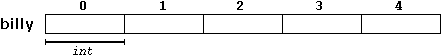where each blank panel represents an element of the array, that in this case are integer values of type int. These elements are numbered from 0 to 4 since in arrays the first index is always 0, independently of its length.

Like a regular variable, an array must be declared before it is used. A typical declaration for an array in C++ is:

type name [elements];

where type is a valid type (like int, float...), name is a valid identifier and the elements field (which is always enclosed in square brackets []), specifies how many of these elements the array has to contain.

Therefore, in order to declare an array called billy as the one shown in the above diagram it is as simple as:

 `` `` ``int billy ;``

NOTE: The elements field within brackets [] which represents the number of elements the array is going to hold, must be a constant value, since arrays are blocks of non-dynamic memory whose size must be determined before execution. In order to create arrays with a variable length dynamic memory is needed, which is explained later in these tutorials.

### Initializing arrays.

When declaring a regular array of local scope (within a function, for example), if we do not specify otherwise, its elements will not be initialized to any value by default, so their content will be undetermined until we store some value in them. The elements of global and static arrays, on the other hand, are automatically initialized with their default values, which for all fundamental types this means they are filled with zeros.

In both cases, local and global, when we declare an array, we have the possibility to assign initial values to each one of its elements by enclosing the values in braces { }. For example:

 `` `` ``int billy  = { 16, 2, 77, 40, 12071 }; ``

This declaration would have created an array like this: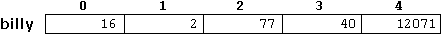The amount of values between braces { } must not be larger than the number of elements that we declare for the array between square brackets [ ]. For example, in the example of array billy we have declared that it has 5 elements and in the list of initial values within braces { } we have specified 5 values, one for each element.

When an initialization of values is provided for an array, C++ allows the possibility of leaving the square brackets empty [ ]. In this case, the compiler will assume a size for the array that matches the number of values included between braces { }:

 `` `` ``int billy [] = { 16, 2, 77, 40, 12071 };``

After this declaration, array billy would be 5 ints long, since we have provided 5 initialization values.

### Accessing the values of an array.

In any point of a program in which an array is visible, we can access the value of any of its elements individually as if it was a normal variable, thus being able to both read and modify its value. The format is as simple as:

name[index]

Following the previous examples in which billy had 5 elements and each of those elements was of type int, the name which we can use to refer to each element is the following: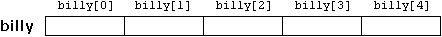For example, to store the value 75 in the third element of billy, we could write the following statement:

 `` `` ``billy = 75;``

and, for example, to pass the value of the third element of billy to a variable called a, we could write:

 `` `` ``a = billy;``

Therefore, the expression billy is for all purposes like a variable of type int.

Notice that the third element of billy is specified billy, since the first one is billy, the second one is billy, and therefore, the third one is billy. By this same reason, its last element is billy. Therefore, if we write billy, we would be accessing the sixth element of billy and therefore exceeding the size of the array.

In C++ it is syntactically correct to exceed the valid range of indices for an array. This can create problems, since accessing out-of-range elements do not cause compilation errors but can cause runtime errors. The reason why this is allowed will be seen further ahead when we begin to use pointers.

At this point it is important to be able to clearly distinguish between the two uses that brackets [ ] have related to arrays. They perform two different tasks: one is to specify the size of arrays when they are declared; and the second one is to specify indices for concrete array elements. Do not confuse these two possible uses of brackets [ ] with arrays.

 ``12`` ``````int billy; // declaration of a new array billy = 75; // access to an element of the array. ``````

If you read carefully, you will see that a type specifier always precedes a variable or array declaration, while it never precedes an access.

Some other valid operations with arrays:

 ``1234`` ``````billy = a; billy[a] = 75; b = billy [a+2]; billy[billy[a]] = billy + 5;``````

 ``12345678910111213141516`` ``````// arrays example #include using namespace std; int billy [] = {16, 2, 77, 40, 12071}; int n, result=0; int main () { for ( n=0 ; n<5 ; n++ ) { result += billy[n]; } cout << result; return 0; }`````` `12206`

### Multidimensional arrays

Multidimensional arrays can be described as "arrays of arrays". For example, a bidimensional array can be imagined as a bidimensional table made of elements, all of them of a same uniform data type.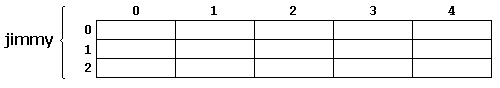jimmy represents a bidimensional array of 3 per 5 elements of type int. The way to declare this array in C++ would be:

 `` `` ``int jimmy ;``

and, for example, the way to reference the second element vertically and fourth horizontally in an expression would be:

 `` `` ``jimmy``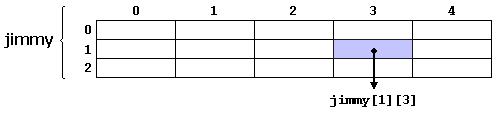(remember that array indices always begin by zero).

Multidimensional arrays are not limited to two indices (i.e., two dimensions). They can contain as many indices as needed. But be careful! The amount of memory needed for an array rapidly increases with each dimension. For example:

 `` `` ``char century ;``

declares an array with a char element for each second in a century, that is more than 3 billion chars. So this declaration would consume more than 3 gigabytes of memory!

Multidimensional arrays are just an abstraction for programmers, since we can obtain the same results with a simple array just by putting a factor between its indices:

 ``12`` ``````int jimmy ; // is equivalent to int jimmy ; // (3 * 5 = 15) ``````

With the only difference that with multidimensional arrays the compiler remembers the depth of each imaginary dimension for us. Take as example these two pieces of code, with both exactly the same result. One uses a bidimensional array and the other one uses a simple array:

multidimensional arraypseudo-multidimensional array
```#define WIDTH 5
#define HEIGHT 3

int jimmy [HEIGHT][WIDTH];
int n,m;

int main ()
{
for (n=0;n<HEIGHT;n++)
for (m=0;m<WIDTH;m++)
{
jimmy[n][m]=(n+1)*(m+1);
}
return 0;
}
```
```#define WIDTH 5
#define HEIGHT 3

int jimmy [HEIGHT * WIDTH];
int n,m;

int main ()
{
for (n=0;n<HEIGHT;n++)
for (m=0;m<WIDTH;m++)
{
jimmy[n*WIDTH+m]=(n+1)*(m+1);
}
return 0;
}
```

None of the two source codes above produce any output on the screen, but both assign values to the memory block called jimmy in the following way: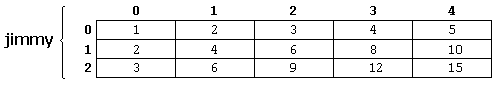We have used "defined constants" (#define) to simplify possible future modifications of the program. For example, in case that we decided to enlarge the array to a height of 4 instead of 3 it could be done simply by changing the line:

 `` `` ``#define HEIGHT 3 ``

to:
 `` `` ``#define HEIGHT 4 ``

with no need to make any other modifications to the program.

### Arrays as parameters

At some moment we may need to pass an array to a function as a parameter. In C++ it is not possible to pass a complete block of memory by value as a parameter to a function, but we are allowed to pass its address. In practice this has almost the same effect and it is a much faster and more efficient operation.

In order to accept arrays as parameters the only thing that we have to do when declaring the function is to specify in its parameters the element type of the array, an identifier and a pair of void brackets []. For example, the following function:

 `` `` ``void procedure (int arg[])``

accepts a parameter of type "array of int" called arg. In order to pass to this function an array declared as:

 `` `` ``int myarray ;``

it would be enough to write a call like this:

 `` `` ``procedure (myarray);``

Here you have a complete example:

 ``123456789101112131415161718`` ``````// arrays as parameters #include using namespace std; void printarray (int arg[], int length) { for (int n=0; n

As you can see, the first parameter (int arg[]) accepts any array whose elements are of type int, whatever its length. For that reason we have included a second parameter that tells the function the length of each array that we pass to it as its first parameter. This allows the for loop that prints out the array to know the range to iterate in the passed array without going out of range.

In a function declaration it is also possible to include multidimensional arrays. The format for a tridimensional array parameter is:

 `` `` ``base_type[][depth][depth]``

for example, a function with a multidimensional array as argument could be:

 `` `` ``void procedure (int myarray[])``

Notice that the first brackets [] are left empty while the following ones specify sizes for their respective dimensions. This is necessary in order for the compiler to be able to determine the depth of each additional dimension.

Arrays, both simple or multidimensional, passed as function parameters are a quite common source of errors for novice programmers. I recommend the reading of the chapter about Pointers for a better understanding on how arrays operate.Previous:Functions (II)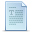IndexNext:Character Sequences